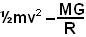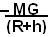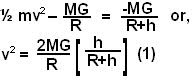## Friday, October 3, 2008

### Irodov Solution 1.221Suppose that the body travels a height h before it starts it journey downwards and let the radius of the Earth be R. We shall solve the problem using conservation of energy.

At the instant when a body starts to move upwards, it total energy is the sum of its kinetic energy and its gravitational potential energy and is given by. When the body reaches its highest point, its has no kinetic energy and its potential energy is given by. So we have,Now if the acceleration of at the surface due to gravity is g, then we have,